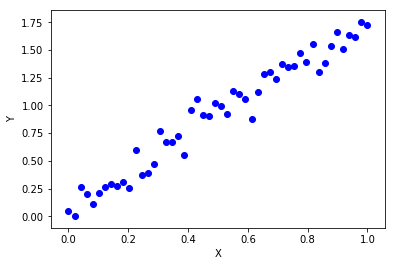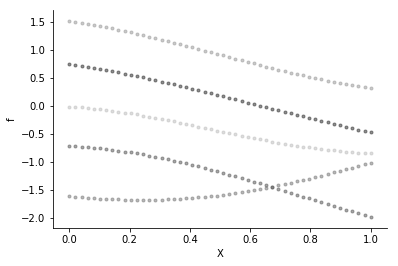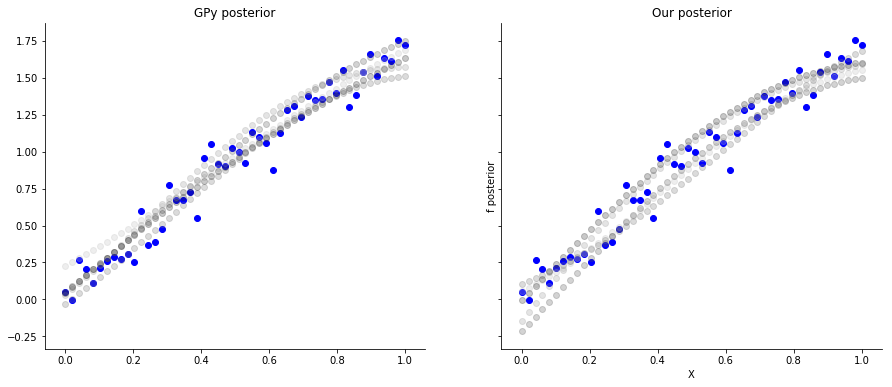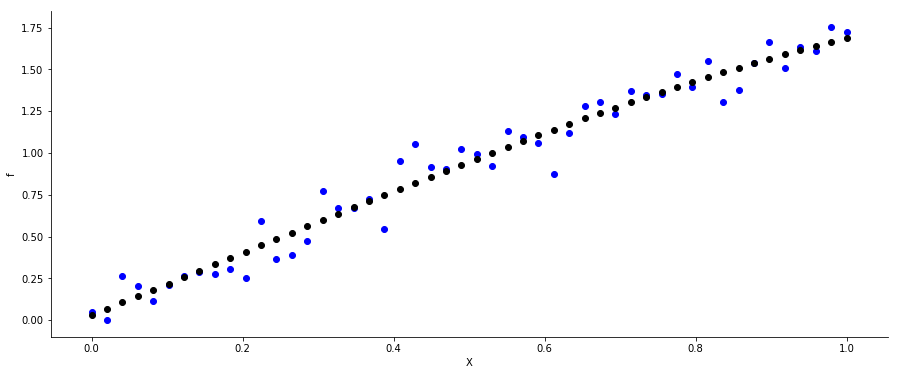# Gaussian process regression¶

Bayesian linear regression derived linear regression in a Bayesian context. Here, we discuss Gaussian process regression using GPy and scipy. Most of the material is from Rasmussen and Williams (2006). I also recommend Michael Betancourt's Robust Gaussian Processes in Stan as a resource, for instance to learn more about hyperparameter inference which won't be covered here.

In :
import GPy
import scipy
from sklearn.metrics.pairwise import rbf_kernel

%matplotlib inline
import matplotlib.pyplot as plt
plt.rcParams['figure.figsize'] = [15, 6]

In :
rnorm = scipy.stats.norm.rvs
mvrnorm = scipy.stats.multivariate_normal.rvs


### Priors on functions¶

In Bayesian linear regression we assumed a linear dependency

\begin{align*} f_{\boldsymbol \beta}& : \ \mathcal{X} \rightarrow \mathcal{Y},\\ f_{\boldsymbol \beta}(\mathbf{x}) & = \ \mathbf{x}^T \boldsymbol \beta + \epsilon, \end{align*}

which was parametrized by a coefficient vector $\boldsymbol \beta$. In order to model uncertainty, regularize our coeffiencts, or what reason whatsoever, we put a prior distribution on $\boldsymbol \beta$ and by that introduced some prior belief to the model.

When we use Gaussian Processes, we instead set a prior on the function $f$ itself:

\begin{align*} f(\mathbf{x}) & \sim \mathcal{GP}(m(\mathbf{x}), k(\mathbf{x}, \mathbf{x}')) ,\\ p(f \mid \mathbf{x}) & = \mathcal{N}(m(\mathbf{x}), k(\mathbf{x}, \mathbf{x}')) . \end{align*}

So a Gaussian process is a distribution of functions. It is parametrized by a mean function $m$ that returns a vector of length $n$ and a kernel function $k$ that returns a matrix of dimension $n \times n$, where $n$ is the number of samples. For instance, the mean function could be a constant (which we will assume throughout the rest of this notebook), and the kernel could be a radial basis function, i.e.:

\begin{align*} m(\mathbf{x}) &= \mathbf{0},\\ k(\mathbf{x}, \mathbf{x}') &= \exp\left(- \gamma ||\mathbf{x} - \mathbf{x}' ||^2 \right), \end{align*}

where $\gamma$ a hyperparameter we have to optimize.

The parameters $\mathbf{m}$ and $\mathbf{k}$ apparently do not have a fixed dimensionality as in Bayesian linear regression (where we had $\boldsymbol \beta \in \mathbb{R}^p$), but have possibly infinite dimension. That means with more data, the dimensions of $\mathbf{m}$ and $\mathbf{k}$ increase (in Bayesian regression $\boldsymbol \beta$ was independent of the sample size $n$). For that reason we call this approach non-parametric (the turn itself sounds confusing, because we apparently have parameters).

Next, let's look at some prior functions $f$. A prior function is just a sample from a $n$-dimensional multivariate normal distribution with mean $\mathbf{m}$ and variance $\mathbf{k}$. The kernel must be postive definite, which the RBF kernel is.

In :
n = 50
x = scipy.linspace(0, 1, n).reshape((n, 1))
beta = 2
y = scipy.sin(x) * beta + rnorm(size=(n, 1), scale=.1)

In :
plt.scatter(x, y, color="blue")
plt.xlabel("X")
plt.ylabel("Y")
plt.show()Then we set the mean and covariance functions.

In :
m = scipy.zeros(n)
kernel = GPy.kern.RBF(input_dim=1)

In :
k = kernel.K(x, x)


Then we sample five functions from the Gaussian process:

In :
f_prior = [mvrnorm(mean=m, cov=k) for i in range(5)]


...and we plot the five samples.

In :
colors = ['#bdbdbd','#969696','#737373','#525252','#252525']
_, ax = plt.subplots()
for i in range(5):
ax.scatter(x, f_prior[i], color=colors[i], marker=".", alpha=0.5)
ax.spines['top'].set_visible(False)
ax.spines['right'].set_visible(False)
plt.xlabel("X")
plt.ylabel("f")
plt.show()This does not look like our data set at all. The reason is that we did not consider the responses $\mathbf{y}$ in the model. We do this by multiplying the prior with the likelihood, which gives us the posterior Gaussian process.

### Posterior Gaussian process¶

We haven't specified the likelihood yet which we will assume to be Gaussian:

\begin{align*} p(\mathbf{y} \mid f, \mathbf{x}) = \prod_i^n \mathcal{N}(f_i, \sigma^2 \mathbf{I} ). \end{align*}

Later, for classification, we will also use a binomial likelihood.

The posterior Gaussian process is given by

\begin{align*} \text{posterior} \propto \text{likelihood} \times \text{prior}. \end{align*}

It is easy to derive the posterior from a joint distribution of the actual observations $\mathbf{y}$ and the posterior function values:

\begin{align*} \left[ \begin{array}{c} \mathbf{y} \\ {f} \end{array} \right] \sim \mathcal{N} \left( \mathbf{0}, \begin{array}{cc} k(\mathbf{x}, \mathbf{x}') + \sigma^2 \mathbf{I} & k(\mathbf{x}, {\mathbf{x}}') \\ k({\mathbf{x}}, \mathbf{x}') & k({\mathbf{x}}, {\mathbf{x}}') \end{array} \right). \end{align*}

Thus conditioning on $\mathbf{y}$ gives:

\begin{align*} p(f \mid \mathbf{y}, \mathbf{x}) & \propto p(\mathbf{y} \mid f, \mathbf{x}) \ p(f \mid \mathbf{x}),\\ f \mid \mathbf{y}, \mathbf{x} & \sim \mathcal{GP}\left(\tilde{m}(\tilde{\mathbf{x}}), \tilde{k}({\mathbf{x}}, {\mathbf{x}}')\right),\\\\ \tilde{m}({\mathbf{x}}) & = k({\mathbf{x}}, \mathbf{x}')\left( k(\mathbf{x}, \mathbf{x}') + \sigma^2 \mathbf{I} \right)^{-1} \mathbf{y},\\ \tilde{k}({\mathbf{x}}, {\mathbf{x}}') & = k({\mathbf{x}}, {\mathbf{x}}') - k({\mathbf{x}}, \mathbf{x}') \left( k(\mathbf{x}, \mathbf{x}') + \sigma^2 \mathbf{I} \right)^{-1} k(\mathbf{x}, {\mathbf{x}}') \end{align*}

So the posterior is again a Gaussian process with modified mean and variance functions. Let's compute this analytically and then compare it to the GPy posterior.

In :
inv = scipy.linalg.inv(k + .1 * scipy.diag(scipy.ones(n)))
m_tilde = (k.dot(inv).dot(y)).flatten()
k_tilde = k - k.dot(inv).dot(k)


Sample from the posterior:

In :
f_posterior = [mvrnorm(mean=m_tilde, cov=k_tilde) for i in range(5)]


In GPy this is way easier, because we only need to call a single function:

In :
m = GPy.models.GPRegression(x, y, kernel, noise_var=.1)
gpy_f_posterior = m.posterior_samples_f(x, full_cov=True, size=5)


Let's compare our posterior to the one from GPy.

In :
plt.rcParams['figure.figsize'] = [15, 6]
colors = ['#bdbdbd','#969696','#737373','#525252','#252525']
_, ax = plt.subplots(1, 2, sharex=True, sharey=True)
ax.scatter(x, y, color="blue")
ax.scatter(x, y, color="blue")
for i in range(5):
ax.scatter(x, gpy_f_posterior[:, i], color=colors[i], alpha=0.25)
ax.scatter(x, f_posterior[i], color=colors[i], alpha=0.25)
ax.spines['top'].set_visible(False)
ax.spines['right'].set_visible(False)
ax.set_title("GPy posterior")
ax.spines['top'].set_visible(False)
ax.spines['right'].set_visible(False)
ax.set_title("Our posterior")
plt.xlabel("X")
plt.ylabel("f posterior")
plt.show()The are pretty much similar. However, we cheated a little, because we knew the error variance.

### Posterior predictive¶

We can use the same formalism as above to derive the posterior predictive distribution, i.e. the distribution of function values $f^*$ for new observations $\mathbf{x}^*$. This is useful, when we want to do prediction.

Usually the predictive posterior is given like this:

\begin{align*} p(f^* \mid \mathbf{y}, \mathbf{x}, \mathbf{x}^*) = \int p(f^* \mid f) \ p(f \mid \mathbf{y}, \mathbf{x}), \end{align*}

(where we included $\mathbf{x}$ for clarity). However, since our original data set $\mathbf{y}$ and $f^*$ have a joint normal distribution, we can just use Gaussian conditioning again. Later, when we are using non-normal likelihoods, we will need to come back to this formulation.

We start again by writing down the joint distribution of $\mathbf{y}$ and the unobserved function values $f^*$:

\begin{align*} \left[ \begin{array}{c} \mathbf{y} \\ {f}^* \end{array} \right] \sim \mathcal{N} \left( \mathbf{0}, \begin{array}{cc} k(\mathbf{x}, \mathbf{x}') + \sigma^2 \mathbf{I} & k(\mathbf{x}, {\mathbf{x}}^*) \\ k({\mathbf{x}}^*, \mathbf{x}) & k({\mathbf{x}}^*, {\mathbf{x}^*}') \end{array} \right). \end{align*}

Conditioning on $\mathbf{y}$ yields:

\begin{align*} f^* \mid \mathbf{y}, \mathbf{x}, \mathbf{x}^* & \sim \mathcal{GP}\left({m}^*({\mathbf{x}^*}), {k}^*({\mathbf{x}^*}, {\mathbf{x}^*}')\right),\\\\ {m}^*({\mathbf{x}^*}) & = k({\mathbf{x}^*}, \mathbf{x})\left( k(\mathbf{x}, \mathbf{x}') + \sigma^2 \mathbf{I} \right)^{-1} \mathbf{y},\\ {k}^*({\mathbf{x}^*}, {\mathbf{x}^*}') & = k({\mathbf{x}^*}, {\mathbf{x}^*}') - k({\mathbf{x}^*}, \mathbf{x}) \left( k(\mathbf{x}, \mathbf{x}') + \sigma^2 \mathbf{I} \right)^{-1} k(\mathbf{x}, {\mathbf{x}^*}). \end{align*}

This is the exact same formulation as above, only that we replaced some of the old data with the new data $\mathbf{x}^*$.

### Prediction¶

Now that the theory is established, we optimize the kernel parameters using m.optimize(), such that the appropriately fit the data. Then we predict the responses $\hat{\mathbf{y}}$ using our trained Gaussian process.

It does not make too much sense to predict the already known values $f$, but posterior predictive checks are always a quick way to check model assumptions.

In :
m.optimize()
y_hat = m.predict(x)


... and plot it again.

In :
_, ax = plt.subplots()
ax.scatter(x, y, color="blue")
ax.scatter(x, y_hat, color="black")
ax.spines['top'].set_visible(False)
ax.spines['right'].set_visible(False)
plt.xlabel("X")
plt.ylabel("f")
plt.show()